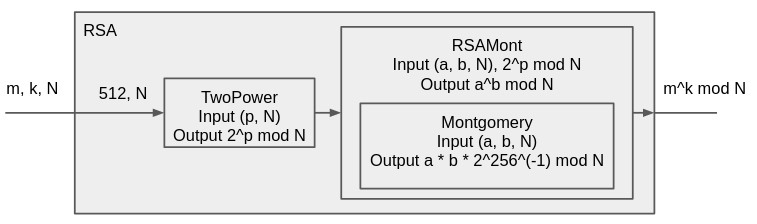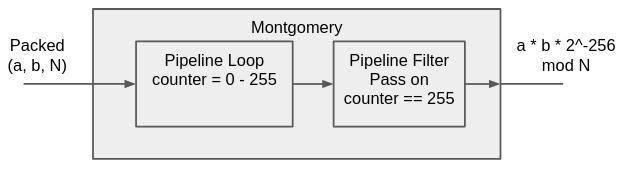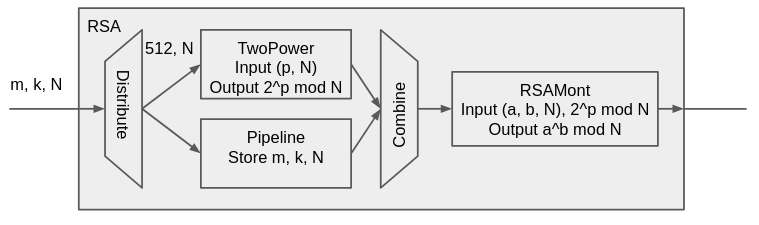# Pipeline#

Module Description
Pipeline 是的這個 module 就叫 pipeline，它控制有 data 要敲一級 Pipeline 這件事，通常會用在一個 module 的最前端，上一級給資料我就先存下來
PipelineFilter 過濾資料，透過外接的 i_pass 訊號，控制輸入資料要不要送去輸出
PipelineDistribute 一對多驅動多個模組，一次驅動後要等待每個模組都收下才會再送下一組，分散的數量透過 parameter 來控制
PipelineCombine 收集來自多個模組的輸入整合為一個輸出，收集的數量透過 parameter 來控制
PipelineLoop 在收到 input 之後，先送出 init signal，接著持續驅動 output module 直到 o_done 訊號為止

Module Description
PipelineDivider 收 N 維的資料，分解成多組 M 維依序輸出，N > M 且 N % M = 0
PipelineMerger 收多組 N 維的資料，組合成 M 維後依序輸出，N < M 且 M % N = 0
PipelineSerializer 收 N 維的資料，切成成多組 M 維，N > M，切到最後會剩下一些剩餘，會跟下一組資料併起來再繼續切斷
PipelineDeserializer 收 N 維的資料，組合成 M 維後輸出，N < M，組合完的剩餘會接在下一組資料上繼續輸出

# RSA256#

• square: $REDC((m \cdot 2^{256} \bmod N) \cdot (m \cdot 2^{256} \bmod N)) = m^2 \cdot 2^{256} \bmod N$
• multiply: $REDC((m \cdot 2^{256} \bmod N) \cdot k) = m \cdot k \bmod N$

## Block Diagram## SystemVerilog Datatype#

parameter MOD_WIDTH = 256;
parameter INT_WIDTH = 32;

typedef logic [MOD_WIDTH-1:0] KeyType;

typedef logic [INT_WIDTH-1:0] IntType;

typedef struct packed {
KeyType a;
KeyType b;
KeyType modulus;
} MontgomeryIn;

typedef KeyType MontgomeryOut;


# Montgomery#

import RSA_pkg::*;

module Montgomery #(
parameter MOD_WIDTH = 256
) (
// input
input clk,
input rst_n,

// input data
input i_valid,
input MontgomeryIn i_in,

// output data
output o_valid,
output MontgomeryOut o_out
);PipelineLoop i_loop(
.clk(clk),
.rst_n(rst_n),
.i_valid(i_valid),
.i_cen(loop_init),
.o_valid(loop_o_valid),
.o_done(loop_done),
.o_cen(loop_next)
);

PipelineFilter i_filter(
.i_valid(loop_o_valid),
.i_pass(loop_done),
.o_valid(o_valid),
);


always_ff @( posedge clk or negedge rst_n ) begin
if (!rst_n) begin
data_a <= 0;
data_b <= 0;
data_modulus <= 0;
end
else begin
if (loop_init) begin
data_a <= {2'b0, i_in.a};
data_b <= {2'b0, i_in.b};
data_modulus <= {2'b0, i_in.modulus};
end
end
end


1. 是要 input 敲 register，combinational circuit 接 output。
2. 還是 output 接 register，input 時先過一層 combinational circuit 再進 register。

round_counter 要在 loop_init 是歸零，loop_next 時遞增。

// round_counter
logic [$clog2(MOD_WIDTH+1)-1:0] round_counter; always_ff @(posedge clk) begin if (loop_init) begin round_counter <= 0; end else if (loop_next) begin round_counter <= round_counter + 1; end end  round_counter 會從 0 數到 255 了，用 round_counter 去取 a 的 bit，計算出要更新的值。 always_comb begin if (loop_done) begin round_result_next = round_result; end else begin round_result_next = round_result; if (data_a[round_counter]) begin round_result_next = round_result + data_b; end if (round_result_next) begin round_result_next += data_modulus; end round_result_next >>= 1; end end  最後像輸出的 combinational circuit 以及 loop_done 都可以用 assign 實作： assign mod_result = round_result > data_modulus ? round_result - data_modulus : round_result; assign o_out = mod_result[MOD_WIDTH - 1 : 0]; logic loop_done = round_counter == MOD_WIDTH;  如此一來就能十分結構化的完成 module 設計，不用明寫整個狀態機，把背後的控制邏輯交給已經驗證過的 pipeline 處理。 ## Pipeline Example# 這裡有另一個例子，說明使用 Pipeline 的好處，在實作 RSA Top module 的時候，曾在打多筆測資時驗到一個 bug。 原因是我在第一級的 pipeline 上把 m, e, N 存下來，N 會立刻送去 TwoPower 計算$ 2^{256} \bmod N $， TwoPower 打包完會跟 m, e, N 整團送去 RSAMont。 而如果 RSA 的 input 擺著下一筆資料要送，就會形成新資料 m’, e’ 蓋掉 m, e 送進 RSAMont，結果當然不是對的。 修正在有 pipeline 下也很簡單，和 TwoPower 並聯一組 Pipeline，前後用 Distribute 和 Combine 連接， 舊資料就可以保存進另一組 register，如下所示。最棒的是因為 distribute 跟 combine 都是同步送與同步收，完全不用擔心該怎麼讓 Pipeline 敲一級 register 這件事， 和 256 cycle 的 TwoPower 要怎麼同步。 順帶一提，在沒用 Pipeline 的設計上，當有一個 module 要花十個 cycle，而有資料要跟它同步時，我是真的看過這樣的寫法： reg data; reg data_w0, data_w1, data_w2, data_w3, data_w4 reg data_w5, data_w6, data_w7, data_w8, data_w9; always @(posedge clk) begin data_w0 <= data; data_w1 <= data_w0; data_w2 <= data_w1; data_w3 <= data_w2; data_w4 <= data_w3; data_w5 <= data_w4; data_w6 <= data_w5; data_w8 <= data_w7; data_w9 <= data_w8; end  這個寫法的擴展性就會很差，假設那個花十個 cycle 的 module 最佳化成 6 個 cycle，這整團修起來就會大費周張。 用上 valid/ready, pipeline 配個 fifo 則根本不會有這個問題，不同速度的 module 可以自動同步。 # Compile and Test# 目前 project 使用 verilator 搭配 verilator cmake 進行測試，設定大概如下： set(LINKME PRIVATE SystemC::systemc glog RSASystemCModel) add_executable(${RSA_SIM} RSA_sim.cpp)
target_link_libraries(${RSA_SIM}${LINKME})
verilate(${RSA_SIM} TRACE_FST PREFIX VRSA SOURCES RSA_pkg.sv RSA.sv) add_test(${RSA_SIM} \${RSA_SIM})